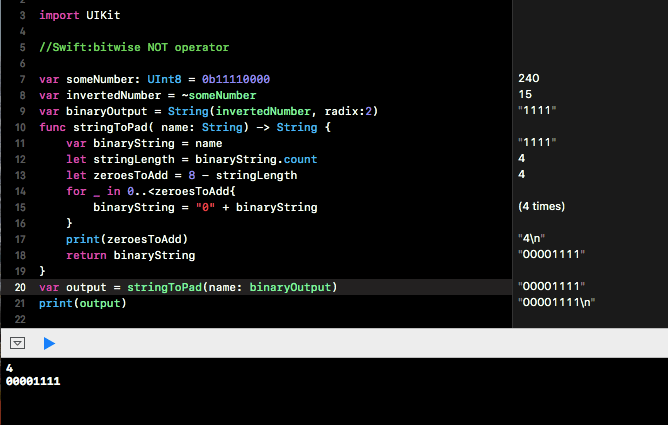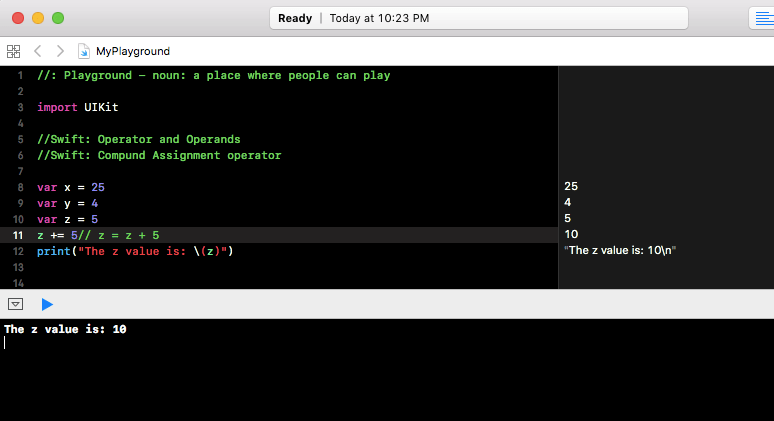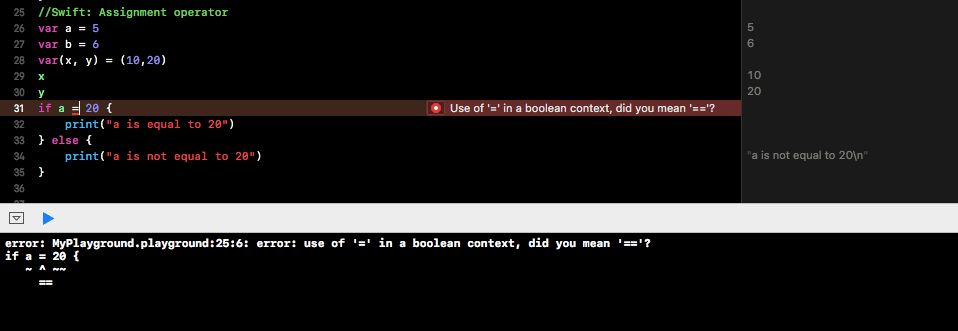# Swift: Type casting for Any and AnyObject

Any can represent an instance of any kind of type and AnyObject can represent an instance of a class type.# Swift: Type Cast operator

A constant or variable may refer to an instance of a subclass behind the scenes. In order to downcast them to their specific subclass , we can use the type cast operator ( as? or as!). As the downcast to a specific subclass may not succeed, the type cast operator comes in two forms. as? returns a optional value that may  or may not be nil. as! operator forcefully unwraps the class type and may result in error if downcasting failed.# Swift: Advanced operator – bitwise XOR operator

Swift provides XOR ( ^ ) operator which compares two numbers and returns a new number in which the bits are set to 1 when the corresponding bits in the numbers are not equal and bits are set to 0 when the bits in those numbers are same.# Swift: Advanced operator – bitwise OR operator

Swift provides bitwise OR ( | ) operator. The OR operator returns a new number with bits set to 1 only when either of the bits present in the two numbers is equal to 1.# Swift: Advanced operator – bitwise AND operator

Swift provides bitwise AND ( & ) operator. The AND operator combines the bits of two numbers and returns a new number with bits set to 1 only when both the bits in those numbers are equal to one.# Swift: Advanced operator – bitwise NOT operator

Swift provides advanced operators such a s bitwise operators. The bitwise NOT ( ~ ) operator inverts all bits in a number.# Swift: Compound Assignment Operator

Compound Assignment Operator combines the assignment operation with other operations. They don’t return a value. It mean the following expression  x = y +=10  can’t be written.# Swift: Assignment operator

Assignment operators in Swift perform two basic functions: Initialise a value or update a value. Consider the example  var a = b + c . Here the assignment operator ‘=’ assigns the value of b+ c to a. Unlike other languages, in Swift the assignment operator will not return a value.  if x = y  is not valid in Swift. When tuples are assigned to variables, they can be decomposed into individual constants and variables as needed.

Swift code:

Output: